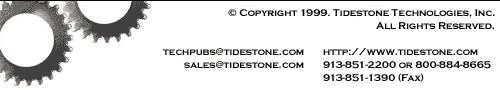# Understanding Cell References

A reference is a cell's address. It identifies a cell or range of cells by referring to the column letter and row number of the cell(s). For example, `A1` refers to the cell at the intersection of column A and row 1.

The reference tells Formula One for Java to use the contents of the referenced cell(s) in the formula. If a formula requires a number, you can substitute a reference to a cell that contains a number.

You specify a range of cells by placing a colon (:) between two cell references. For example, `A1:C3` refers to the range anchored by cells A1 and C3. The range includes all cells in columns A, B, and C of rows 1, 2, and 3.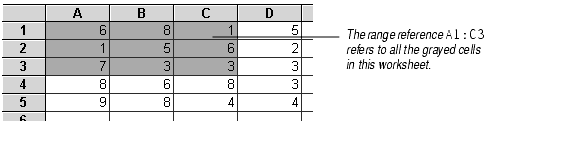# Entering Cell References

You can enter cell references in formulas in three ways:

• Type in the cell or range address.
• Type in the name of a named cell or range. For information on defining names, see Using Names.
• Use the mouse to click and drag on cells and ranges. Formula One for Java automatically enters a relative reference identifying the cell(s) you select.

# Absolute and Relative References

There are two types of cell references: relative and absolute.

• Relative references point to a cell based on its position relative to the current cell. When the cell containing the reference is moved or copied, the reference is adjusted to point to a new cell with the same relative offset as the originally referenced cell.
For example, suppose the formula `=B1+B2+B3` is located in cell A1. When you copy the formula and paste it down two rows into cell A3, the formula will be adjusted down two rows, to `=B3+B4+B5`.
Relative references will be adjusted whenever you cut or copy and paste a formula or when you use the Edit > Fill commands to fill a range with a copy of a formula.
• Absolute references point to a cell at an exact location. When a cell containing a formula with absolute references is moved or copied, the reference does not change. Absolute references have a dollar sign (\$) in front of the row number and/or column letter.

References that are part absolute and part relative are called mixed references. The following table lists the reference types.
Reference Type
A1 Relative reference pointing to cell A1.
\$A\$1 Absolute reference pointing to cell A1.
\$A1 Absolute column reference, relative row reference pointing to cell A1.
A\$1 Relative column reference, absolute row reference pointing to cell A1.

# Using Relative and Absolute References

You can copy and paste absolute, relative, and mixed references to create worksheets that are easy to update and that are smaller than worksheets where each formula is created separately.

For example, in the following worksheet, the values in column A need to be multiplied by the percentages in row 1.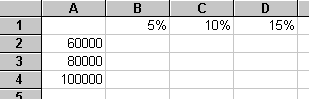To do these calculations, you could enter the formula `=A2*B1` in cell B2, `=A3*B1` in cell B3, `=A4*B1` in cell B4, etc. Besides the fact that this would require a lot of typing, this solution would require Formula One for Java to keep nine separate formulas in memory.

A better way to do it would be to enter the formula `=\$A2*B\$1` in cell B2, and use the worksheet's Edit > Fill command to fill cells B2 through D4 with copies of that formula. When the formula is copied in this manner, its relative references change, but the absolute references stay the same.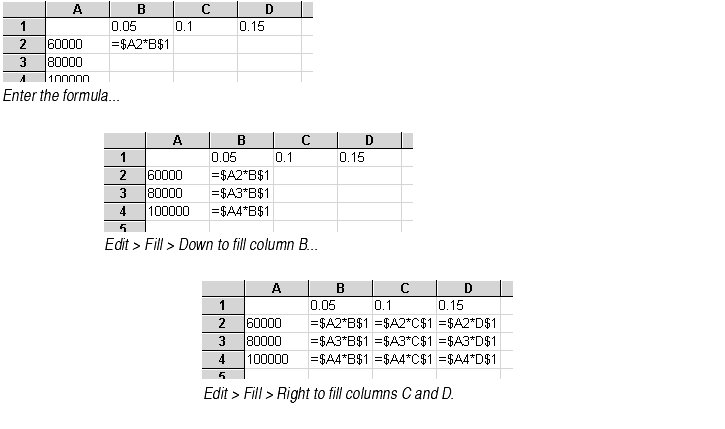The resulting worksheet calculates all the figures using multiple copies of that one formula. Only one formula must be kept in memory.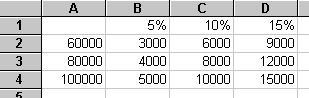If you change the percentages in row 1 or the figures in column A, the calculations in the worksheet will automatically change because of the absolute references to those cells. This makes the worksheet easy to update.

# References to Other Worksheets

You can reference cells in other worksheets in the same workbook by placing an exclamation mark between the sheet name and the reference. The sheet name is the name found on the worksheet tab. For example, `Data!A1` refers to the top left cell in a sheet called Data.

Sheet names with spaces. If the sheet name contains spaces, you must enclose the name in single quotes: ``1994 sales'!B17`.

Cells on two worksheets. You can make a reference to cells on two different worksheets by placing a colon between the two sheet names. For example, Sheet1:Sheet2!A1 refers to two cells: cell A1 in Sheet1 and cell A1 in Sheet2.

Order of sheet names. References to more than one worksheet must list the worksheets in the order in which they appear in the workbook.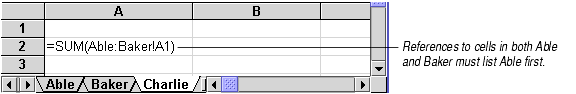# References to Other Workbooks

References that point to cells on worksheets in other workbooks are called external references.

An external reference is created by placing the workbook name in brackets, followed by the worksheet name, an exclamation point, and finally a cell or range reference.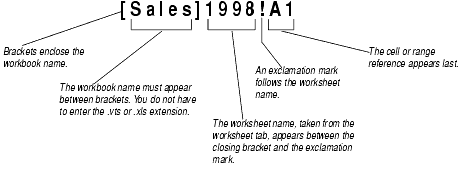External references will work only if both workbooks are open in the Workbook Designer. If the referenced workbook is not open when you create the external reference, an Invalid Formula Syntax error message will appear.

The following are examples of external references using absolute, relative, and mixed references.
Reference Type
[Sales]1987!A1 Relative reference pointing to cell A1 in a worksheet titled 1987 of a workbook titled Sales.
[FY91]January!\$A\$1 Absolute reference pointing to cell A1 in a worksheet titled January of a workbook titled FY91.
[Q1]Sheet1:Sheet2!\$A1 Absolute column reference, relative row reference pointing to cell A1 in the first and second worksheets of a workbook titled Q1.
[Store1]Sheet1:Sheet4!A1:F1 Relative row and column reference pointing to the range A1 to F1 in the first four worksheets of a workbook titled Store1.

## Paths in External References

After you enter an external reference, Formula One for Java will change the format of the reference to show the absolute path to the workbook you referenced. For example, say you entered this reference to a workbook named September in the Payroll directory on your C drive:

[September]Payroll!C2:C420

After you enter that reference, if you return to the cell where the reference was entered, you will note that Formula One for Java has changed it to:

`[C:\Payroll\September.vts]Payroll'!C2:C420

This absolute path is recorded in the worksheet. If you later move the September workbook, the external reference should still work, as long as you open September.vts in the Workbook Designer at the same time as the workbook that references it.